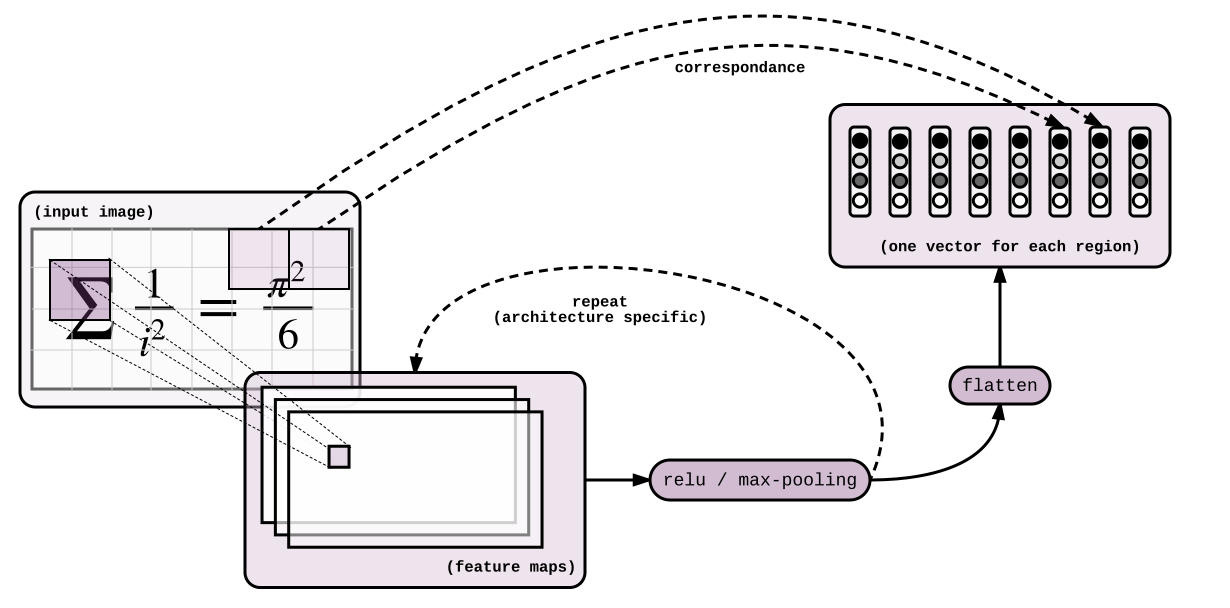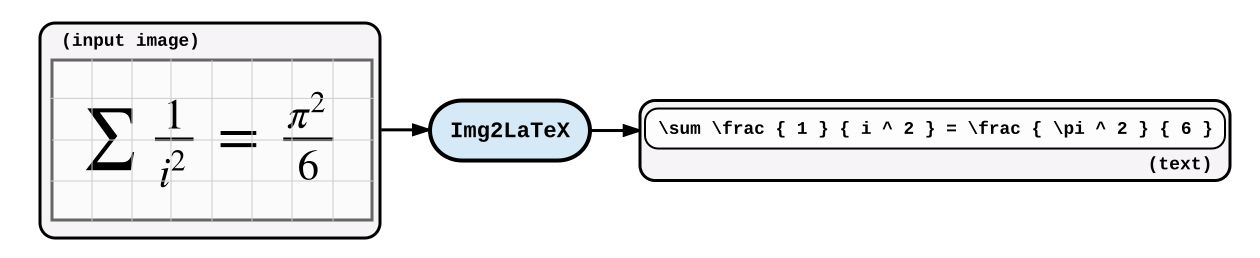## 基于Seq2Seq的图像latex生成

2018年08月12日

1. 如何将seq2seq模型应用于LaTex生成
2. 如何使用Tensorflow实现

## 数据

\alpha + \beta
\frac { 1 } { 2 }
\frac { \alpha } { \beta }
1 + 2


0.png 0
1.png 1
2.png 2
3.png 3


+ 1 2 \alpha \beta \frac { }


## 模型

# batch of images, shape = (batch size, height, width, 1)
img = tf.placeholder(tf.uint8, shape=(None, None, None, 1), name='img')
# batch of formulas, shape = (batch size, length of the formula)
formula = tf.placeholder(tf.int32, shape=(None, None), name='formula')
formula_length = tf.placeholder(tf.int32, shape=(None, ), name='formula_length')


### 编码器

High-level idea 在图片上应用卷积网络，输出一个向量序列$[e_{1},… e_{n}]$对应输入图像的相应部分。这些向量将会对应于翻译部分的LSTM的隐层状态。# casting the image back to float32 on the GPU
img = tf.cast(img, tf.float32) / 255.

out = tf.layers.conv2d(img, 64, 3, 1, "SAME", activation=tf.nn.relu)
out = tf.layers.max_pooling2d(out, 2, 2, "SAME")

out = tf.layers.conv2d(out, 128, 3, 1, "SAME", activation=tf.nn.relu)
out = tf.layers.max_pooling2d(out, 2, 2, "SAME")

out = tf.layers.conv2d(out, 256, 3, 1, "SAME", activation=tf.nn.relu)

out = tf.layers.conv2d(out, 256, 3, 1, "SAME", activation=tf.nn.relu)
out = tf.layers.max_pooling2d(out, (2, 1), (2, 1), "SAME")

out = tf.layers.conv2d(out, 512, 3, 1, "SAME", activation=tf.nn.relu)
out = tf.layers.max_pooling2d(out, (1, 2), (1, 2), "SAME")

# encoder representation, shape = (batch size, height', width', 512)
out = tf.layers.conv2d(out, 512, 3, 1, "VALID", activation=tf.nn.relu)


H, W = tf.shape(out)[1:2]
seq = tf.reshape(out, shape=[-1, H*W, 512])-_-below can discuss!-_-